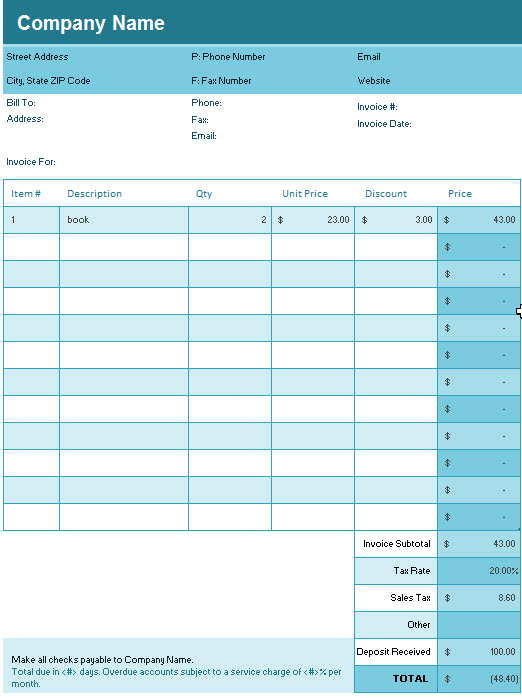# Simple Invoice Template In MS Excel

This invoice template for excel will calculate line item totals, sales tax, Deposit Received and deduct discount offered.  And it is very easy to customize and use. And you can download it freely to use it in your business.### Simple invoice Templates Description

Invoice Subtotal: This excel invoice template will calculate the subtotal amount automatically in Cell G21, and it call one SUM function (=SUM(SimpleInvoice[Price])) in this excel cell.

Sales Tax: If you do not want to calculate the Sales Tax, just leave “TaxRate” as blank. Or it will convert the value to percentage format.

TOTAL:  The Total Amount will be calculated automatically in Cell G26 using excel Formula: (G21+G23+G24)-G25